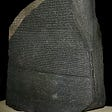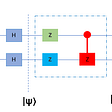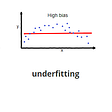# Simple linear regression model

• Predict whether a tumor is malignant or benign, in real-estate domain, weather predictions, and so on..lets dive into the issue :)

## the simple linear regressionfunction:

• we start by importing the libraries and the dataset, and drop empty training examples
• next we try to visualize the data
• we get the data visualization above , now lets observe the relationship between the data that is the x and y components of the training example
• the graph below shows how correlated the values are
• we then build our linear regression model using the linear regression function in scikit learn
• we then determine the accuracy of our model by simply testing it with the test data
• our model predict values with an 98.88% accuracy, pretty good huh !
• So that was a small tutorial for newbies in the domain and want to embrace the world of machine learning i hope it was intresting, stay tuned for more tips

--

--

--

## More from Nken Allassan

deep learning and datascience enthusiast

Love podcasts or audiobooks? Learn on the go with our new app.

## Recurrent Neural Networks for Language Translation## INTRODUCTION TO MACHINE LEARNING## Quantum Circuit## Building a Neural Language Model with tf.eager## Spectral Feature of Graph Signals## Week 3: More interesting experiments## Nken Allassan

deep learning and datascience enthusiast

## Evaluation of Classification Model## The Only Linear Regression Explanation You Need## Walking Through Training Models